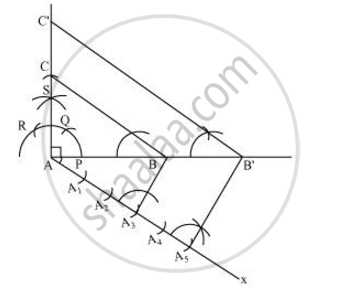# Draw a right triangle in which the sides (other than hypotenuse) are of lengths 4 cm and 3 cm. the construct another triangle whose sides are 5/3 times the corresponding sides of the given triangle. Give the justification of the construction. - Mathematics

Draw a right triangle in which the sides (other than hypotenuse) are of lengths 4 cm and 3 cm. the construct another triangle whose sides are 5/3 times the corresponding sides of the given triangle. Give the justification of the construction.

#### Solution

It is given that sides other than hypotenuse are of lengths 4 cm and 3 cm. Clearly, these will be perpendicular to each other.

The required triangle can be drawn as follows.

Step 1

Draw a line segment AB = 4 cm. Draw a ray SA making 90° with it.

Step 2

Draw an arc of 3 cm radius while taking A as its centre to intersect SA at C. Join BC. ΔABC is the required triangle.

Step 3

Draw a ray AX making an acute angle with AB, opposite to vertex C.

Step 4

Locate 5 points (as 5 is greater in 5 and 3), A1, A2, A3, A4, A5, on line segment AX such that AA1 = A1A2= A2A3 = A3A4 = A4A5.

Step 5

Join A3B. Draw a line through A5 parallel to A3B intersecting extended line segment AB at B'.

Step 6

Through B', draw a line parallel to BC intersecting extended line segment AC at C'. ΔAB'C' is the required triangle.Justification

The construction can be justified by proving that

AB' =5/3 AB, B'C' = 5/3 BC, AC' = 5/3 AC

In ΔABC and ΔAB'C',

∠ABC = ∠AB'C' (Corresponding angles)

∠BAC = ∠B'AC' (Common)

∴ ΔABC ∼ ΔAB'C' (AA similarity criterion)

=> (AB)/(AB')=(BC)/(B'C') = (AC)/(AC') ...1

In ΔAA3B and ΔAA5B',

∠A3AB = ∠A5AB' (Common)

∠AA3B = ∠AA5B' (Corresponding angles)

∴ ΔAA3B ∼ ΔAA5B' (AA similarity criterion)

=> (AB)/(AB') = (

=>(AB)/(AB') = 3/5 ....2

On comparing equations (1) and (2), we obtain

(AB)/(AB') = (BC)/(B'C') = (AC)/(AC') = 3/5

=> AB' =5/3 AB, B'C' = 5/3 BC, AC' = 5/3 AC

This justifies the construction.

Concept: Division of a Line Segment
Is there an error in this question or solution?
Chapter 11: Constructions - Exercise 11.1 [Page 220]

#### APPEARS IN

NCERT Class 10 Maths
Chapter 11 Constructions
Exercise 11.1 | Q 7 | Page 220

Share